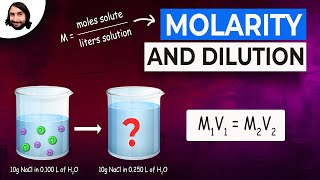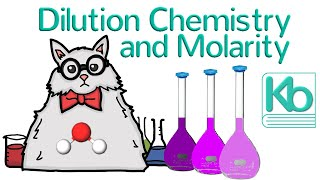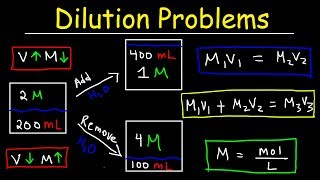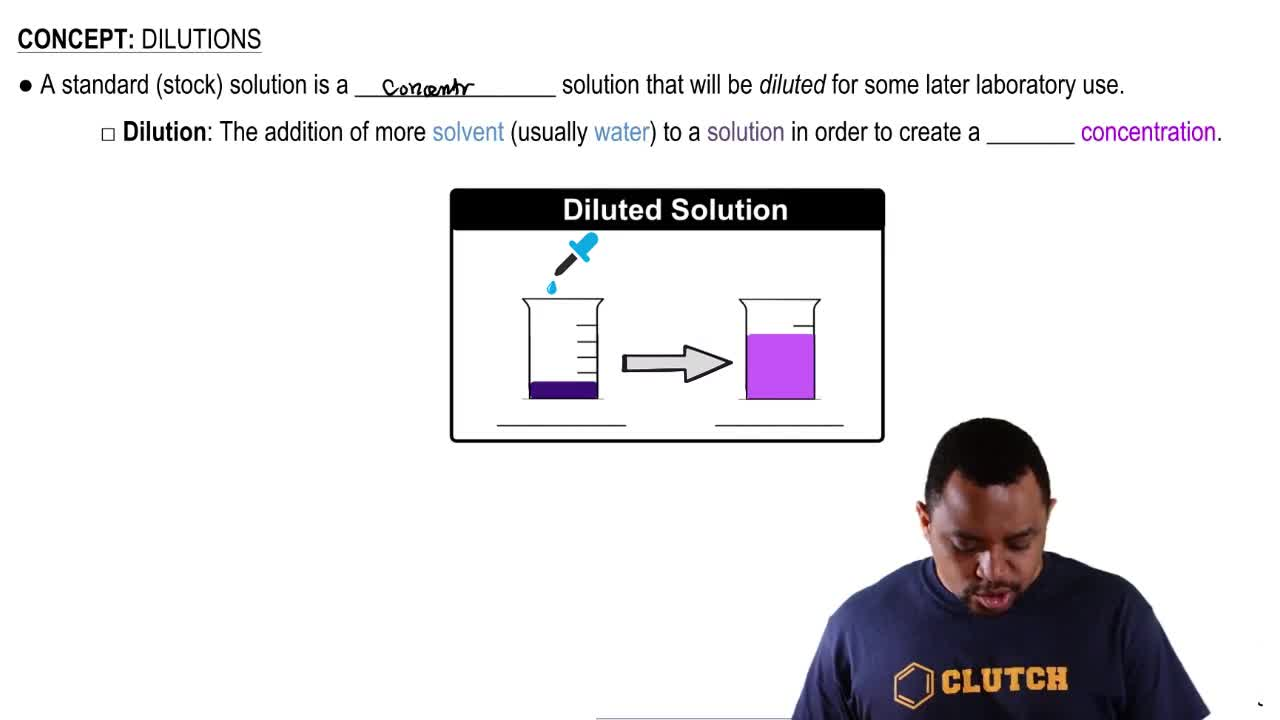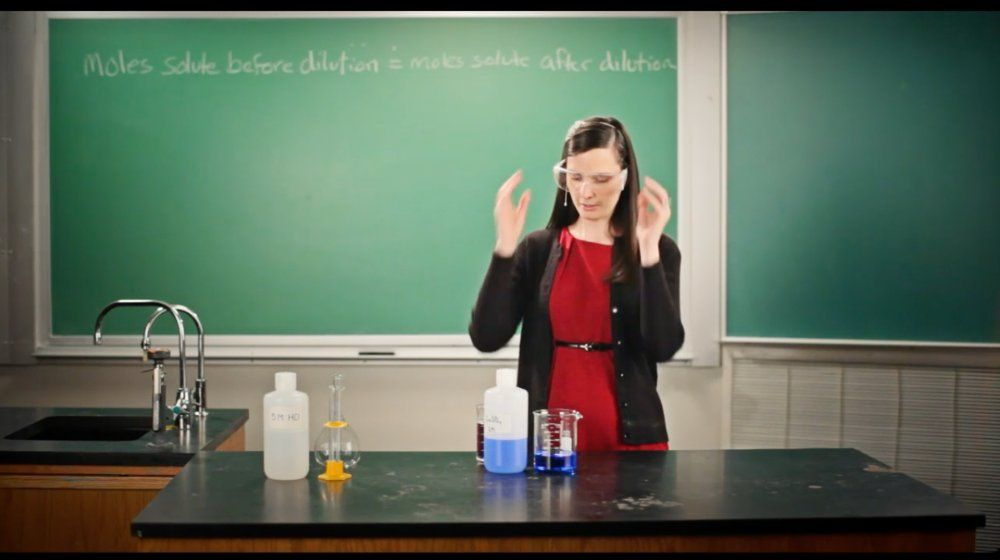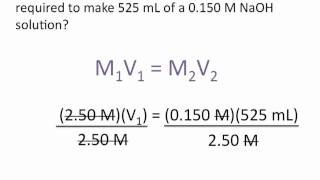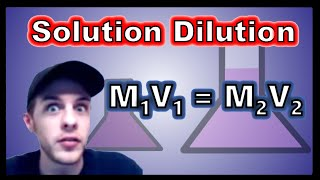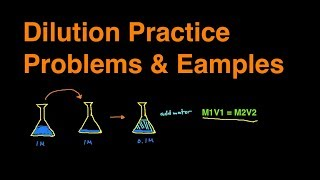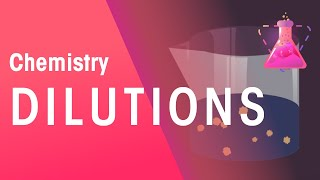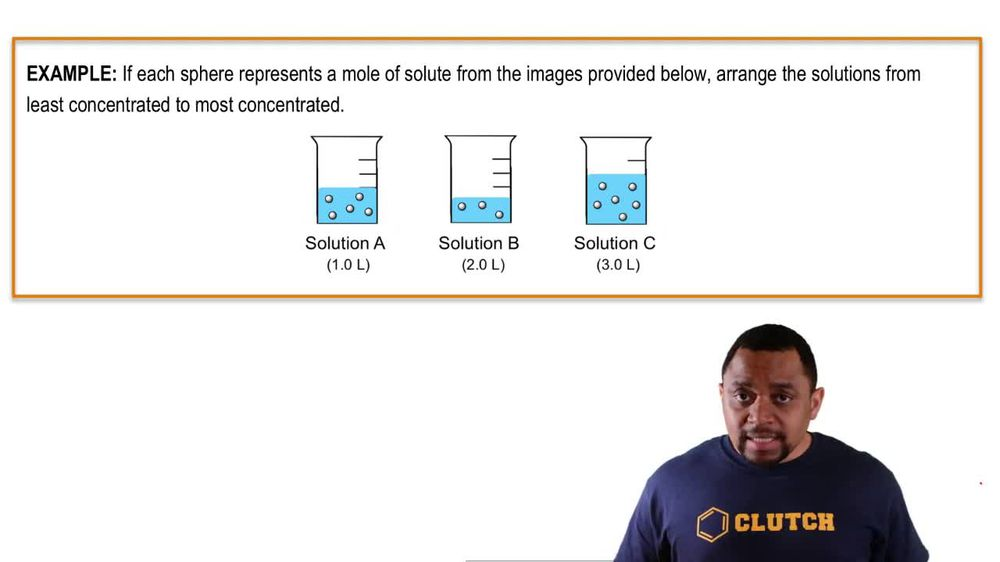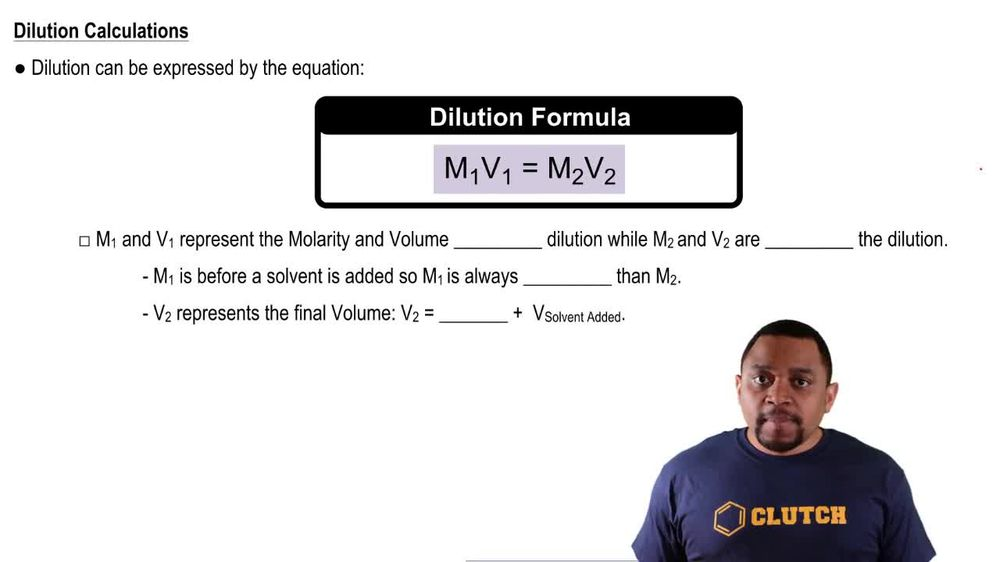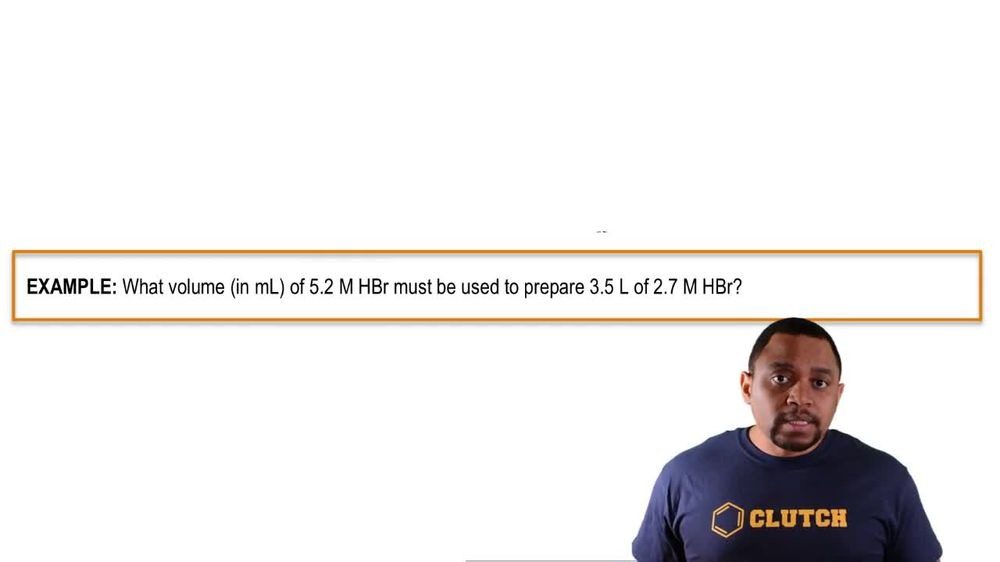Start typing, then use the up and down arrows to select an option from the list.
1. 6. Chemical Quantities & Aqueous Reactions2. Dilutions# Dilutions

by Jules Bruno
175 views
0
So at this point we know that a dilution makes our solutions less concentrated. It takes us from a larger, more clarity value toe a smaller polarity value. We're gonna say dilution can be expressed by the following equation. So it's M one V one equals M two V to hear M one and V one represent the polarity and volume before dilution. While M two and V two are after the dilution, we're going to stay here. M one is before a solvent has added So m one which is the more concentrated solution is always larger than M two, which will be the diluted solution. Now the two represents your final volume. And how exactly did we get to be too? Well, we started out with an initial volume and we added water to it. So the two equals the one plus the volume of solvent added